# 前向传播算法(Forward propagation)与反向传播算法(Back propagation)

## 1.前向传播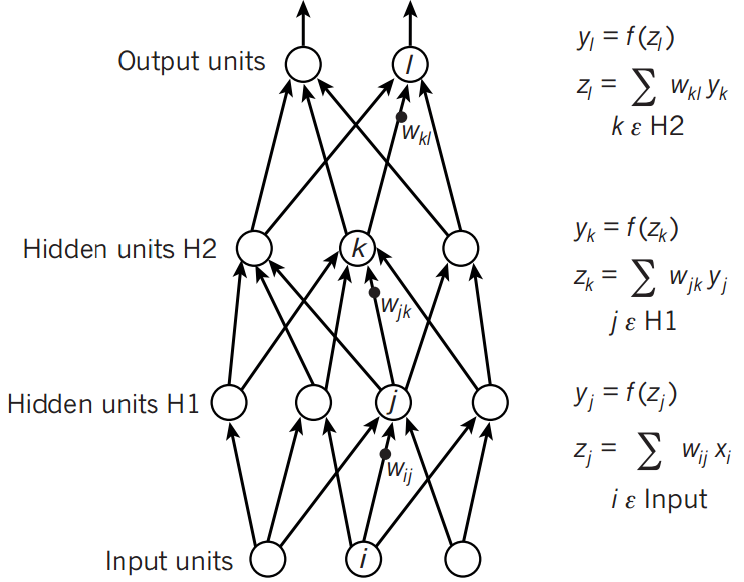a2=σ(z2)=σ(a1W2+b2)

## 2.反向传播算法(Back propagation)

BackPropagation算法是多层神经网络的训练中举足轻重的算法。简单的理解，它的确就是复合函数的链式法则，但其在实际运算中的意义比链式法则要大的多。要回答题主这个问题“如何直观的解释back propagation算法？” 需要先直观理解多层神经网络的训练。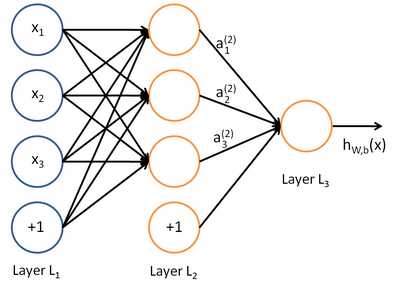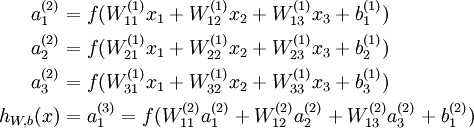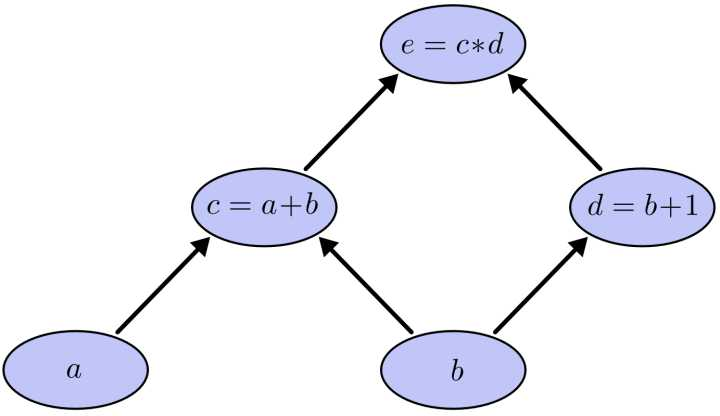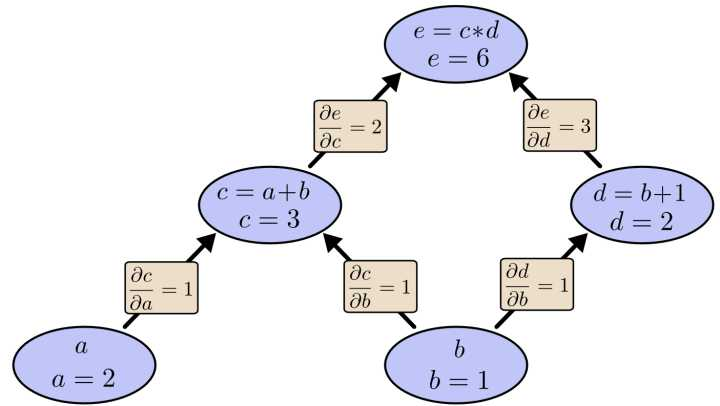ea=eccaeb=eccb+eddb

## 3.反向传播具体计算过程推导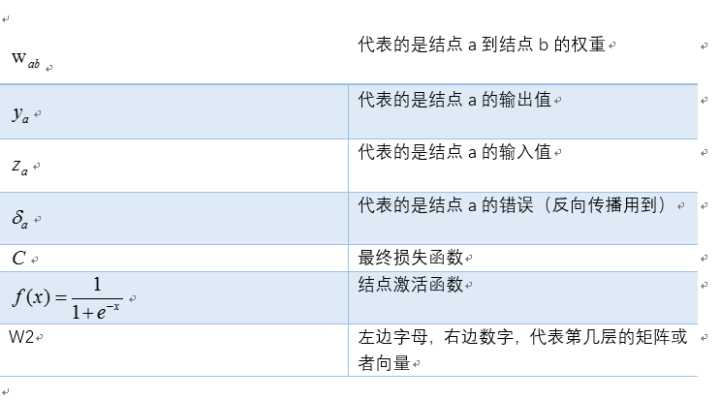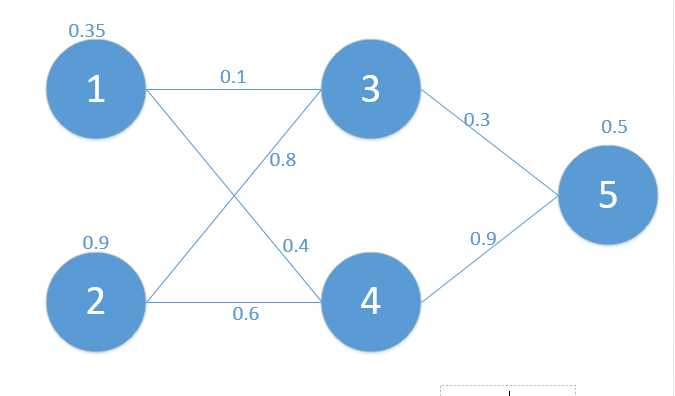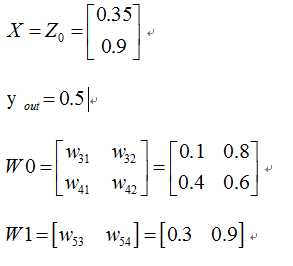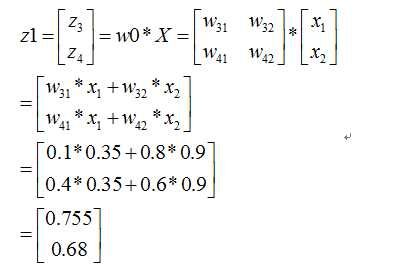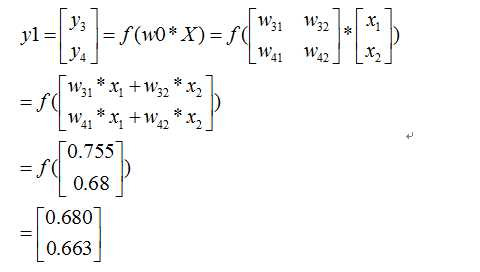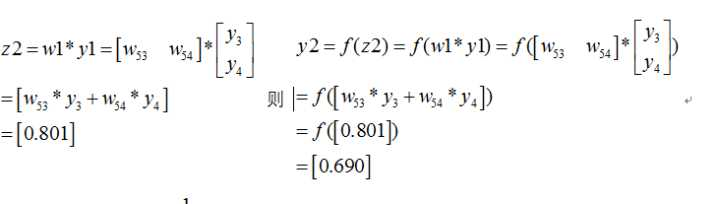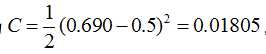，我们当然是希望这个值越小越好。这也是我们为什么要进行训练，调节参数，使得最终的损失最小。这就用到了我们的反向传播算法，实际上反向传播就是梯度下降法中链式法则的使用。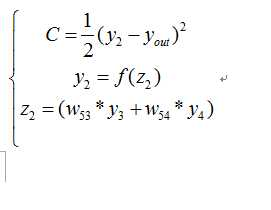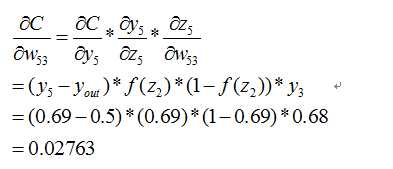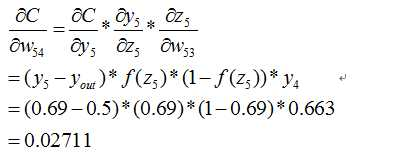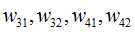，下面给出一个推导其它全都类似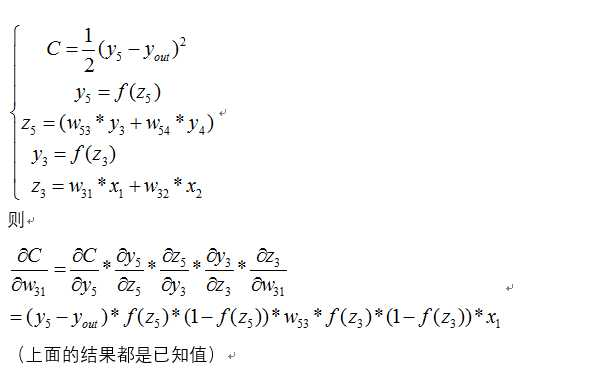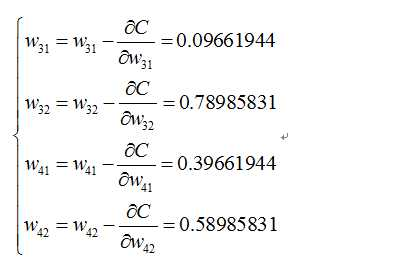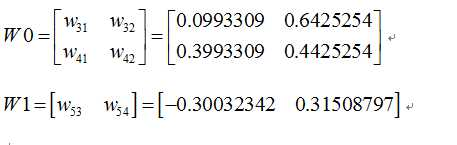bp过程可能差不多就是这样了，可能此文需要你以前接触过bp算法，只是还有疑惑，一步步推导后，会有较深的理解。

#!/usr/bin/env python
#coding:utf-8

import numpy as np

def nonlin(x, deriv = False):
if(deriv == True):
return x * (1 - x)
return 1 / (1 + np.exp(-x))

X = np.array([[0.35], [0.9]])
y = np.array([[0.5]])

np.random.seed(1)

W0 = np.array([[0.1, 0.8], [0.4, 0.6]])
W1 = np.array([[0.3, 0.9]])

print 'original ', W0, '\n', W1

for j in xrange(100):
l0 = X
l1 = nonlin(np.dot(W0, l0))
l2 = nonlin(np.dot(W1, l1))
l2_error = y - l2
Error = 1 / 2.0 * (y-l2)**2
print 'Error:', Error

l2_delta = l2_error * nonlin(l2, deriv=True)

l1_error = l2_delta * W1 #back propagation
l1_delta = l1_error * nonlin(l1, deriv=True)

W1 += l2_delta * l1.T
W0 += l0.T.dot(l1_delta)
print W0, '\n', W1